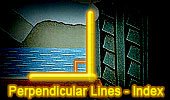Perpendicular Lines, Theorems and Problems - Table of Content  (16)Proposed Problem 273. Triangle, Perpendicular lines, Parallel lines, Area of Squares. Proposed Problem 252. Rectangle, isosceles right triangle, Perpendicular lines, square, area. Proposed Problem 251. Parallelogram, sides, diagonals, squares, Perpendicular lines, areas. Eight-Point Circle Theorem Step-by-Step construction, Manipulation, and animation. TracenPoche Interactive Geometry Software Perpendicular diagonals. Simson Line. using Tracenpoche Interactive Geometry Software. Step-by-Step construction, Manipulation, and animation. Perpendicular diagonals. Triangle: Incircle, Perpendicular, Angle Bisector. Using Tracenpoche Interactive Geometry Software. Step-by-Step construction, Manipulation, and animation Perpendicular diagonals. Orthopole of a Triangle Using TracenPoche Dynamic Geometry Software Perpendicular diagonals. Proposed Problem 237. Quadrilateral, Midpoints, Exterior line, Perpendicular lines, Parallel lines. Proposed Problem 236. Quadrilateral, Midpoints, Exterior line, Perpendicular lines, Parallel lines. Proposed Problem 235. Parallelogram, Transversal line, Perpendicular lines, Parallel lines. Proposed Problem 234. Parallelogram, Line through a vertex, Perpendicular lines, Parallel lines. Proposed Problem 233. Parallelogram, Exterior line, Perpendicular lines, Parallel lines. Proposed Problem 232. Parallelogram, Line through a vertex, Perpendicular lines. Proposed Problem 231. Triangle, Midpoints, Transversal, Perpendicular lines, Parallel lines. Go to Page: Previous | 1 | 2 | 3 | 4 | 5 | 6 | 7 | 8 | 9 | 10 | 11 | 12 | 13 | 14 | 15 | 16 | 17 | 18 | 19 | 20 | Next

 Home | Search | Geometry | Post a comment | Email | Post a comment | By Antonio Gutierrez Last updated: Feb 15, 2015